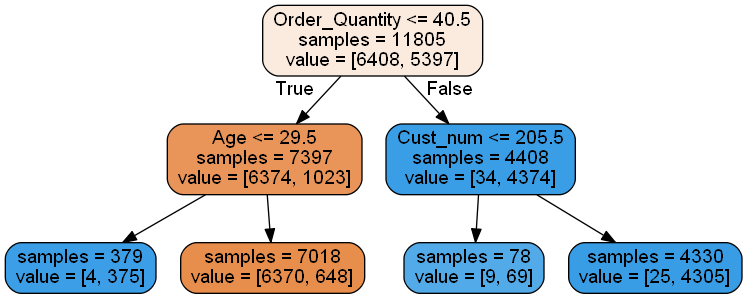• No products in the cart.

204.3.7 Building a Decision Tree in Python

Building a Decision Tree, finding major characteristics and plotting the tree.

Link to the previous post : https://statinfer.com/204-3-6-the-decision-tree-algorithm/

Here comes the fun part of this series. Building a decision tree in python and plotting the same. But, before that let us have a recap of how the decision tree algorithm works.

The Decision tree Algorithm- Full version

Until stopped:

1. Select a leaf node
2. Select an attribute
• Partition the node population and calculate information gain.
• Find the split with maximum information gain for this attribute
3. Repeat this for all attributes
• Find the best splitting attribute along with best split rule
4. Spilt the node using the attribute
5. Go to each child node and repeat step 2 to 4

Stopping criteria:

• Each leaf-node contains examples of one type
• Algorithm ran out of attributes
• No further significant information gain

Practice : Decision Tree Building

We don’t need to take care of each step, python package Sci-kit has a pre-built API to take care of it, we just need to feed the parameters.

• Import Data:Ecom_Cust_Relationship_Management/Ecom_Cust_Survey.csv
• How many customers have participated in the survey?
• Overall most of the customers are satisfied or dis-satisfied?
• Can you segment the data and find the concentrated satisfied and dis-satisfied customer segments ?
• What are the major characteristics of satisfied customers?
• What are the major characteristics of dis-satisfied customers?

Solution

#Import Data
import pandas as pd

# to remove all the missing values rows
df.dropna(inplace='True')
#Q 1. How many customers have participated in the survey?
df.shape
#ANS: 11805
(11805, 7)
#total number of customers rows
df.shape
11805
#Q.2 Overall most of the customers are satisfied or dis-satisfied?
df.Overall_Satisfaction.value_counts()
Dis Satisfied    6408
Satisfied        5397
Name: Overall_Satisfaction, dtype: int64
#number of satisfied customers
satisfied = df['Overall_Satisfaction'].map( {'Dis Satisfied': 0, 'Satisfied': 1} ).astype(int).sum()
satisfied
5397
#number of dis satisfied customers
df.shape-satisfied
#ANS: 6411
6408
df.Overall_Satisfaction.value_counts()
Dis Satisfied    6408
Satisfied        5397
Name: Overall_Satisfaction, dtype: int64
• Can you segment the data and find the concentrated satisfied and dis-satisfied customer segments ?

We will create a tree model in python using the sci-kit module. Before that we will need to convert most of the feature data into numerical or hash values as scikit only works with numerical data.

# Welcome to variable transformation
df['Region'] = df['Region'].map( {'EAST': 1, 'WEST': 2, 'NORTH': 3, 'SOUTH':4} ).astype(int)
df['Customer_Type'] = df['Customer_Type'].map({'Prime': 1, 'Non_Prime': 0}).astype(int)
#We will also need to change the column names, as '.' and spaces are part of many basic funcions in python
df.rename(columns={'Order Quantity':'Order_Quantity', 'Improvement Area' :'Improvement_Area'}, inplace=True)

df['Improvement_Area'] = df['Improvement_Area'].map({'Website UI':1, 'Packing & Shipping':2, 'Product Quality':3,}).astype(int)
df['Overall_Satisfaction'] = df['Overall_Satisfaction'].map( {'Dis Satisfied': 0, 'Satisfied': 1} ).astype(int)
#Need the library to create the tree
from sklearn import tree

#Defining Features and lables
features= list(df.columns[:6])
X = df[features]
y = df['Overall_Satisfaction']

#Building Tree Model
clf = tree.DecisionTreeClassifier(max_depth=2)
clf.fit(X,y)
DecisionTreeClassifier(class_weight=None, criterion='gini', max_depth=2,
max_features=None, max_leaf_nodes=None, min_samples_leaf=1,
min_samples_split=2, min_weight_fraction_leaf=0.0,
presort=False, random_state=None, splitter='best')

Plotting the trees

Unfortunately drawing a beautiful tree is not easy in python as it is in R, none the less we need a way out.

• Have a latest version of jyputer and python installed, anaconda with python 3.5 and jupyter 4.2.3 is being used in this session.
• We will need to install graphviz tool in our system and set the path in environment variables.
• Get the path for gvedit.exe in install directory(for me it was “C:\Program Files (x86)\Graphviz2.38\bin\”)
• We will need python package pydotplus(for older python versions pydot)
• use this command in your anaconda prompt: conda install -c conda-forge pydotplus
• if an error regarding version occure while installing the package go to https://anaconda.org/search?q=pydotplus
• this link will show the channel name of the suitable version suitable.
• and we can use use : conda install -c <channel name here> pydotplus

note: older version of python uses pydot(depreciated); use pydotplus for new versions

#What are the major characteristics of satisfied customers?
from IPython.display import Image
from sklearn.externals.six import StringIO
import pydotplus
dot_data = StringIO()
tree.export_graphviz(clf,
out_file = dot_data,
feature_names = features,
filled=True, rounded=True,
impurity=False)

graph = pydotplus.graph_from_dot_data(dot_data.getvalue())
Image(graph.create_png())By looking at the plot we can answer two questions:

• What are the major characteristics of satisfied customers?Order.Quantity less than 40.5 and Age less than 29.5 or Order.Quantity greater than equal to 40.5.
• What are the major characteristics of dis-satisfied customers?Order.Quantity more than 40.5 & Age greater than equal to 29.5.

The next post is about practice session on validating the tree.

Link to the next post : https://statinfer.com/204-3-8-practice-validating-the-tree/

0 responses on "204.3.7 Building a Decision Tree in Python"